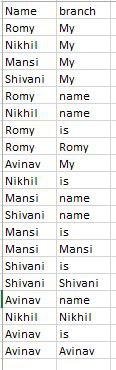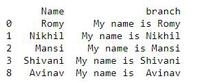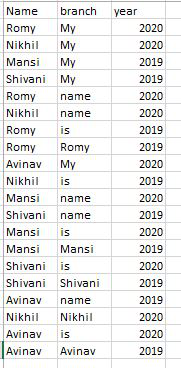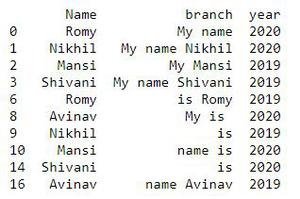# Concatenate strings from several rows using Pandas groupby

• Difficulty Level : Basic
• Last Updated : 20 Aug, 2020

Pandas Dataframe.groupby() method is used to split the data into groups based on some criteria. The abstract definition of grouping is to provide a mapping of labels to the group name.

To concatenate string from several rows using Dataframe.groupby(), perform the following steps:

1. Group the data using Dataframe.groupby() method whose attributes you need to concatenate.
2. Concatenate the string by using the join function and transform the value of that column using lambda statement.

We will use the CSV file having 2 columns, the content of the file is shown in the below image:Example 1: We will concatenate the data in the branch column having the same name.

## Python3

 `# import pandas library``import` `pandas as pd`` ` `# read csv file``df ``=` `pd.read_csv(``"Book2.csv"``)`` ` `# concatenate the string``df[``'branch'``] ``=` `df.groupby([``'Name'``])[``'branch'``].transform(``lambda` `x : ``' '``.join(x))`` ` `# drop duplicate data``df ``=` `df.drop_duplicates()   `` ` `# show the dataframe``print``(df)`

Output:Example 2: We can perform Pandas groupby on multiple columns as well.

We will use the CSV file having 3 columns, the content of the file is shown in the below image:Apply groupby on Name and year column

## Python3

 `# import pandas library``import` `pandas as pd`` ` `# read a csv file``df ``=` `pd.read_csv(``"Book1.csv"``)`` ` `# concatenate the string``df[``'branch'``] ``=` `df.groupby([``'Name'``, ``'year'``])[``'branch'``].transform(``                                              ``lambda` `x: ``' '``.join(x))`` ` `# drop duplicate data``df ``=` `df.drop_duplicates()          `` ` `# show the dataframe``df`

Output:My Personal Notes arrow_drop_up